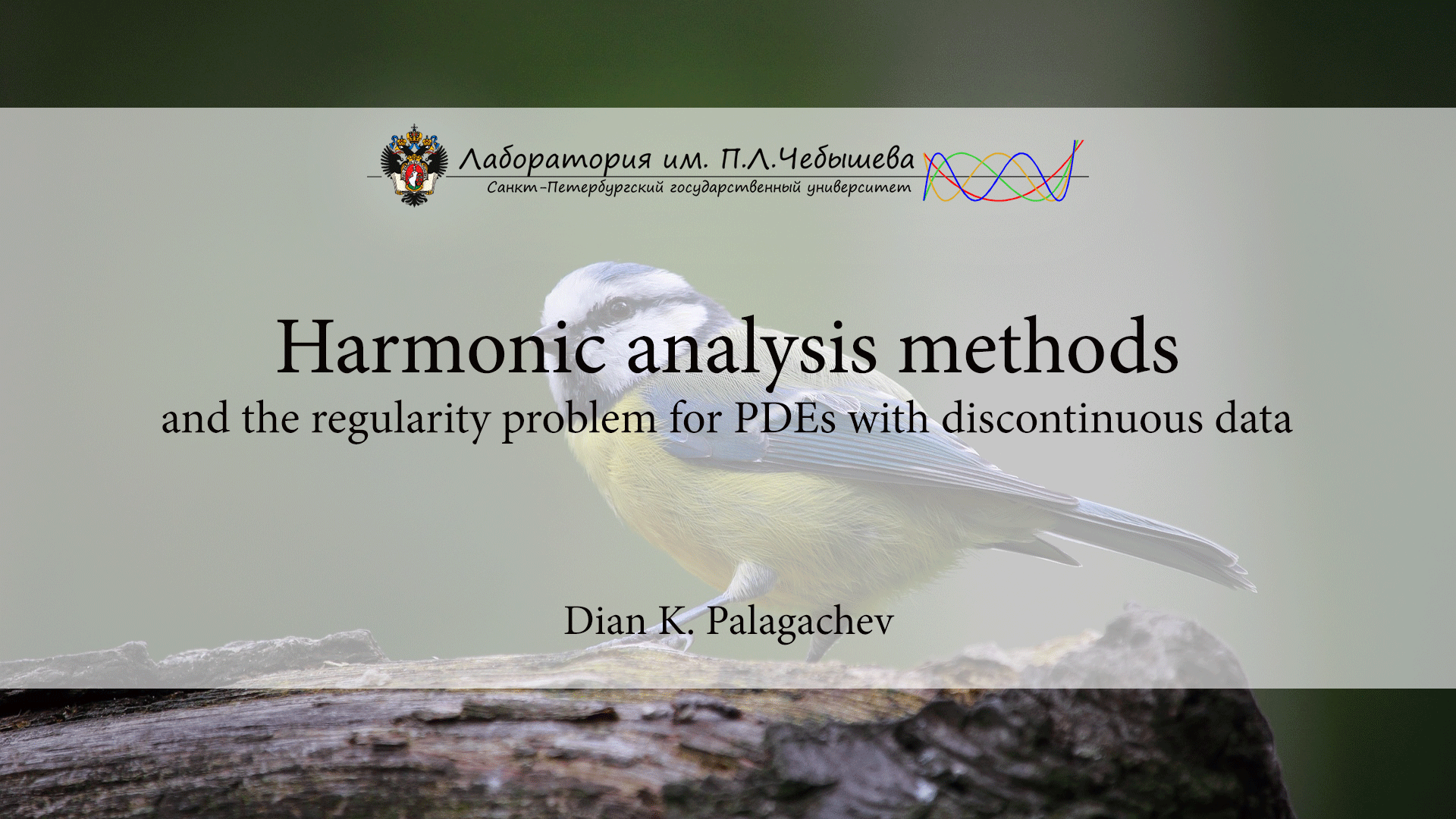# Harmonic analysis methods and the regularity problem for PDEs with discontinuous data

Курс
Предмет:
Лектор:Aims and objectives: The purpose of this course is to provide to the students the modern techniques, based on harmonic analysis methods, to treat the regularity problem for elliptic equations in non-divergence form with discontinuous coefficients.

Prerequisites: Knowledge of the Lebesgue and the Sobolev spaces, Fourier series expansions, Basic functional analysis, Familiarity with the basic properties of uniformly elliptic operators, Aleksandrov maximum principle, Calderón-Zygmund inequality (Sections 9.1 till 9.4 of Gilbarg & Trudinger’s book).

Sillabus:

• Basic facts from the harmonic analysis. Singular integrals of CalderónZygmund type, Hardy-Littlewood’s and sharp maximal operators, Spaces BMO and VMO with examples, The John-Nirenberg lemma.
• L p -boundedness of commutators of singular integrals and the multiplication by a BMO-function. Commutators with Calderón-Zygmund type kernels. Hörmander’s integral condition.
• Applications to the regularity problem for second (and eventually higher) order elliptic equations in non-divergence form. The case of parabolic operators.Representation formulas for the second derivatives of the strong solutions. Reduction to commutators. Interior estimates for the second derivatives. Sketch of the approach to derive boundary estimates for the Dirichlet problem.

4